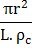# Two wires of same metal have the same length but their cross sections are in the retio 3:1. They are joined in series. The resistance of the thicker wire is. The total resistance of the combination is a)b)c)d)## Question ID - 150321 :- Two wires of same metal have the same length but their cross sections are in the retio 3:1. They are joined in series. The resistance of the thicker wire is. The total resistance of the combination is a)b)c)d)3537

 (c)  For the same length and same material,The resistance of thick wire,The resistance of thin wireTotal resistanceA copper wire of lengthand radiusis nickel plated till its final radius becomebut length remains. If the resistivity of nickel and copper beandrespectively, the conductance of the nickelled wire is a)b)c)d)